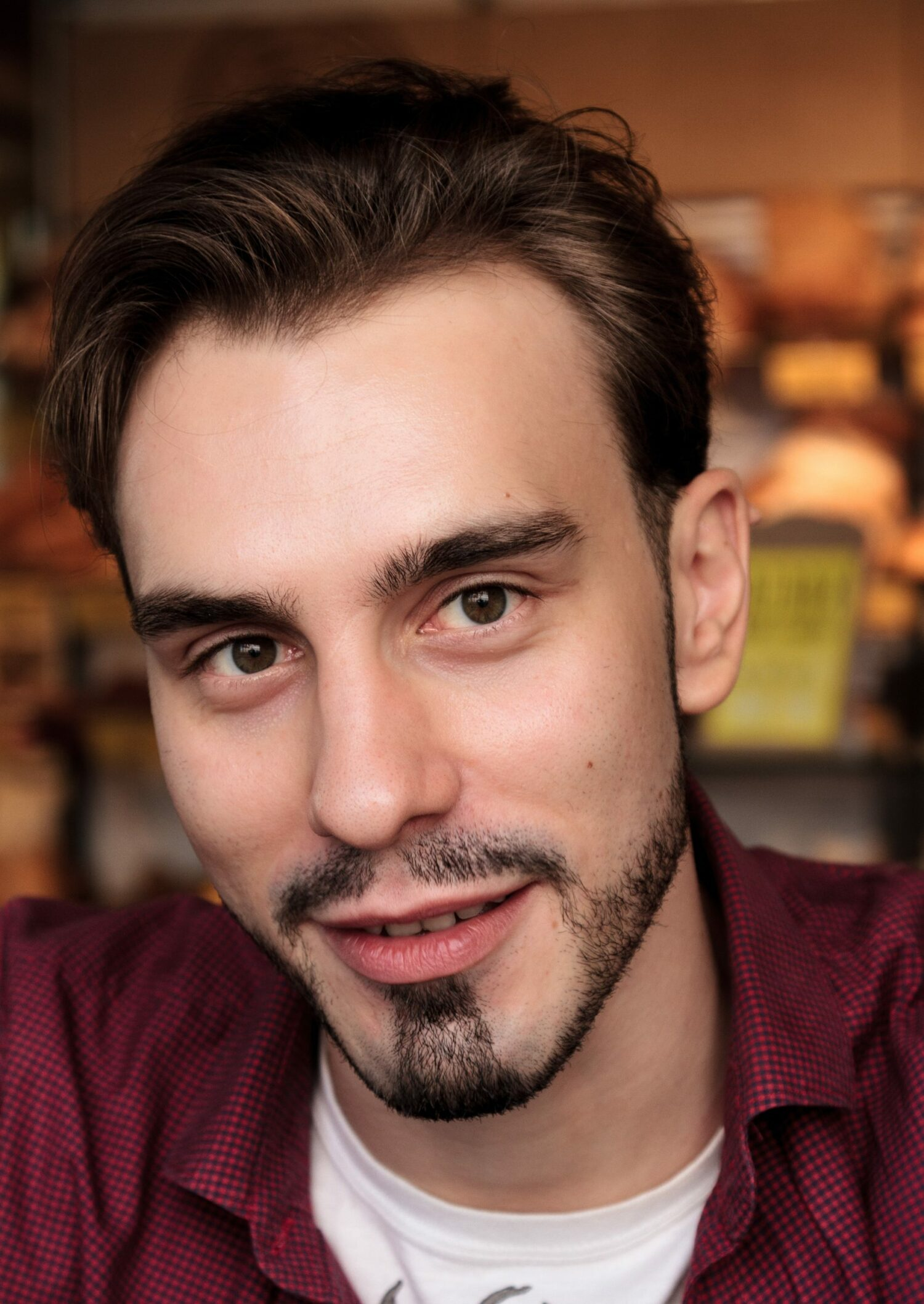Select PageAndrei Lavrenov
Junior Researcher
SPbU
Education:
06.2018 PhD in Mathematical Logic, Algebra and Number Theory (01.01.06)
Institution: St.Petersburg Department of V.A. Steklov Institute of Mathematics of the Russian Academy of Sciences
Thesis title: The structure of Steinberg groups
Supervisor: Nikolai Vavilov

06.2014 Specialist Degree in Mathematics
Institution: SPbU, Faculty of Mathematics and Mechanics, Department of Higher Algebra and Number Theory

The head of the research project "Algebraic Morava K-theory"

Abstract: The theme is devoted to the algebraic Morava K-theory, and to the corresponding category of motives. The category of Chow motives was defined by Grothendieck, and since then have been actively studied. However, the theory still have dozens of open problems. On the other hand, the categories of motives can be constructed by any oriented cohomology theory, and, e.g., the category of K_0-motives is much simpler, but it carries less information about the varieties. Morava K-theories K(n) are the series of oriented cohomology theories starting from K_0, and tending to Chow in a certain sense. We study them to get simpler invariants of varieties, then Chow motives, and at the same time carrying more information, then K_0-motives.
Interests:
Morav K-theory, algebraic groups